### Randomized Complete Block with One Factor

This example illustrates the use of PROC ANOVA in analyzing a randomized complete block design. Researchers are interested in whether three treatments have different effects on the yield and worth of a particular crop. They believe that the experimental units are not homogeneous. So, a blocking factor is introduced that allows the experimental units to be homogeneous within each block. The three treatments are then randomly assigned within each block.

The data from this study are input into the SAS data set `RCB`:

```title1 'Randomized Complete Block';
data RCB;
input Block Treatment \$ Yield Worth @@;
datalines;
1 A 32.6 112   1 B 36.4 130   1 C 29.5 106
2 A 42.7 139   2 B 47.1 143   2 C 32.9 112
3 A 35.3 124   3 B 40.1 134   3 C 33.6 116
;
```

The variables `Yield` and `Worth` are continuous response variables, and the variables `Block` and `Treatment` are the classification variables. Because the data for the analysis are balanced, you can use PROC ANOVA to run the analysis.

The statements for the analysis are

```proc anova data=RCB;
class Block Treatment;
model Yield Worth=Block Treatment;
run;
```

The `Block` and `Treatment` effects appear in the CLASS statement. The MODEL statement requests an analysis for each of the two dependent variables, `Yield` and `Worth`.

Figure 25.5 shows the Class Level Information table.

Figure 25.5: Class Level Information

 Randomized Complete Block

The ANOVA Procedure

Class Level Information
Class Levels Values
Block 3 1 2 3
Treatment 3 A B C

 Number of Observations Read 9 9

The Class Level Information table lists the number of levels and their values for all effects specified in the CLASS statement. The number of observations in the data set are also displayed. Use this information to make sure that the data have been read correctly.

The overall ANOVA table for `Yield` in Figure 25.6 appears first in the output because it is the first response variable listed on the left side in the MODEL statement.

Figure 25.6: Overall ANOVA Table for Yield

 Randomized Complete Block

The ANOVA Procedure

Dependent Variable: Yield

Source DF Sum of Squares Mean Square F Value Pr > F
Model 4 225.2777778 56.3194444 8.94 0.0283
Error 4 25.1911111 6.2977778
Corrected Total 8 250.4688889

R-Square Coeff Var Root MSE Yield Mean
0.899424 6.840047 2.509537 36.68889

The overall F statistic is significant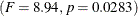, indicating that the model as a whole accounts for a significant portion of the variation in `Yield` and that you can proceed to evaluate the tests of effects.

The degrees of freedom (DF) are used to ensure correctness of the data and model. The Corrected Total degrees of freedom are one less than the total number of observations in the data set; in this case, 9 – 1 = 8. The Model degrees of freedom for a randomized complete block are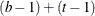, where b = number of block levels and t = number of treatment levels. In this case, this formula leads to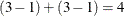model degrees of freedom.

Several simple statistics follow the ANOVA table. The R-Square indicates that the model accounts for nearly 90% of the variation in the variable `Yield`. The coefficient of variation (C.V.) is listed along with the Root MSE and the mean of the dependent variable. The Root MSE is an estimate of the standard deviation of the dependent variable. The C.V. is a unitless measure of variability.

The tests of the effects shown in Figure 25.7 are displayed after the simple statistics.

Figure 25.7: Tests of Effects for Yield

Source DF Anova SS Mean Square F Value Pr > F
Block 2 98.1755556 49.0877778 7.79 0.0417
Treatment 2 127.1022222 63.5511111 10.09 0.0274

For `Yield`, both the `Block` and `Treatment` effects are significant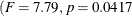and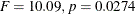, respectively) at the 95% level. From this you can conclude that blocking is useful for this variable and that some contrast between the treatment means is significantly different from zero.

Figure 25.8 shows the ANOVA table, simple statistics, and tests of effects for the variable `Worth`.

Figure 25.8: ANOVA Table for Worth

 Randomized Complete Block

The ANOVA Procedure

Dependent Variable: Worth

Source DF Sum of Squares Mean Square F Value Pr > F
Model 4 1247.333333 311.833333 8.28 0.0323
Error 4 150.666667 37.666667
Corrected Total 8 1398.000000

R-Square Coeff Var Root MSE Worth Mean
0.892227 4.949450 6.137318 124.0000

Source DF Anova SS Mean Square F Value Pr > F
Block 2 354.6666667 177.3333333 4.71 0.0889
Treatment 2 892.6666667 446.3333333 11.85 0.0209

The overall F test is significant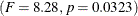at the 95% level for the variable `Worth`. The `Block` effect is not significant at the 0.05 level but is significant at the 0.10 confidence level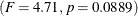. Generally, the usefulness of blocking should be determined before the analysis. However, since there are two dependent variables of interest, and `Block` is significant for one of them (`Yield`), blocking appears to be generally useful. For `Worth`, as with `Yield`, the effect of `Treatment` is significant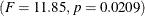.

Issuing the following command produces the `Treatment` means.

```   means Treatment;
run;
```

Figure 25.9 displays the treatment means and their standard deviations for both dependent variables.

Figure 25.9: Means of Yield and Worth

 Randomized Complete Block

The ANOVA Procedure

Level of
Treatment
N Yield Worth
Mean Std Dev Mean Std Dev
A 3 36.8666667 5.22908532 125.000000 13.5277493
B 3 41.2000000 5.43415127 135.666667 6.6583281
C 3 32.0000000 2.19317122 111.333333 5.0332230